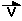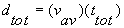Speed is the rate of change of distance with respect to time. The symbol for speed is V and the  base units for speed in the metric system is  [m/s]

Velocity is the vector "equivalent" of speed.  The velocity of a moving object is the speed the object travels in one particular direction.

The symbol for velocity is.

Example To indicate the velocity of a transport truck going at a speed of 100 km/h to Montreal (in an easterly direction) you would write= 100 km/h [E] ---> this is the velocity of the truck!

To calculate the average speed (or average velocity) of an object we use the general equationExample:

If you are walking with your dog in the park at 30 cm/s.  How far will you have traveled in 20 minutes?[tip: don't forget to change units from cm/s to m/s and minutes into seconds][tip: when you're asked to find "how far" it means ... "find the distance"]

Given:  vav = 30 cm/s = 0.30 m/s
t tot =  20 min = 20 min X 60 s/min = 1200 s

Find: dtot  --> use the above equation for vav but rearrange it so that you can solve  for dtot

Solution:dtot = 0.30 m/s  X 1200 s  = 360 m

Therefore you and the dog would have walked a total distance of 360 m